Finding Increase or Decrease Percent

Chapter 8 Class 8 Comparing Quantities
Concept wise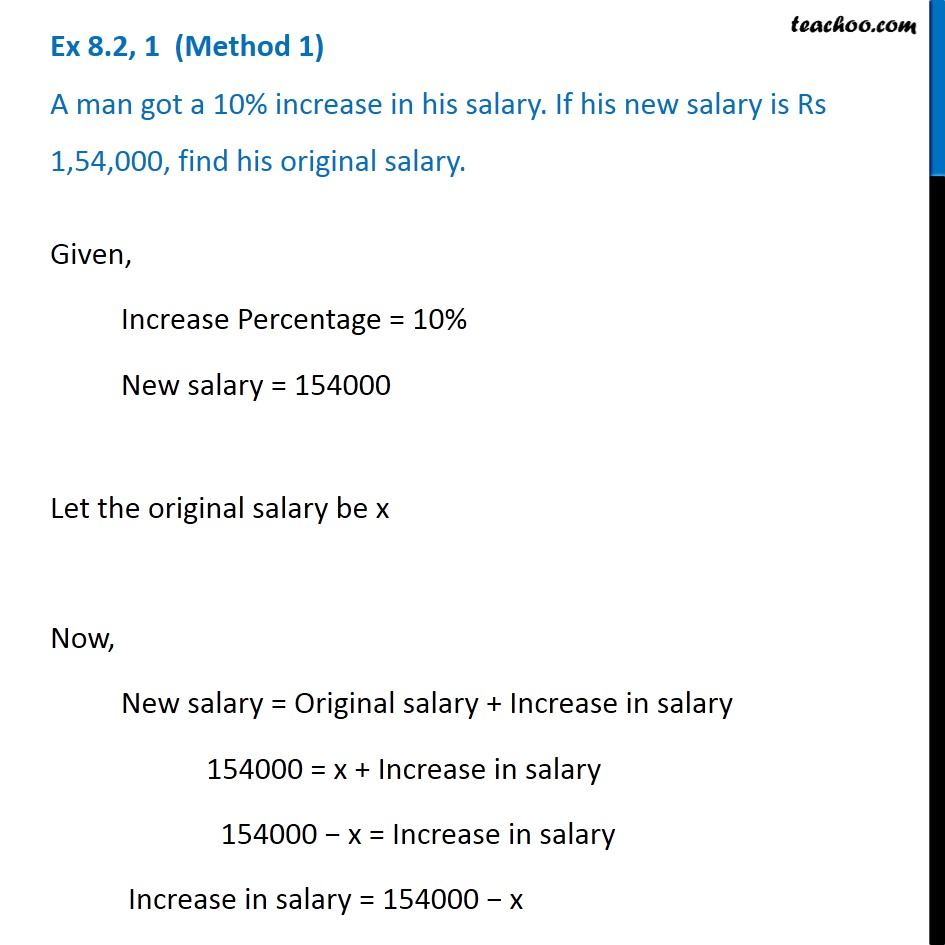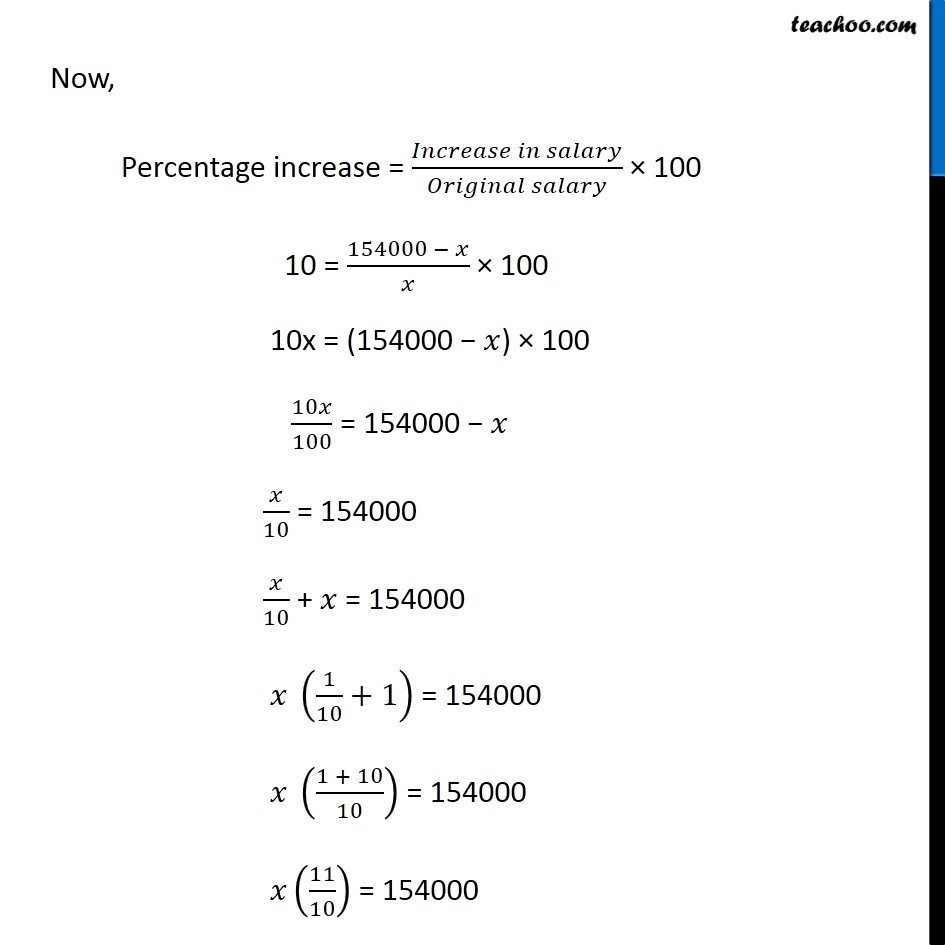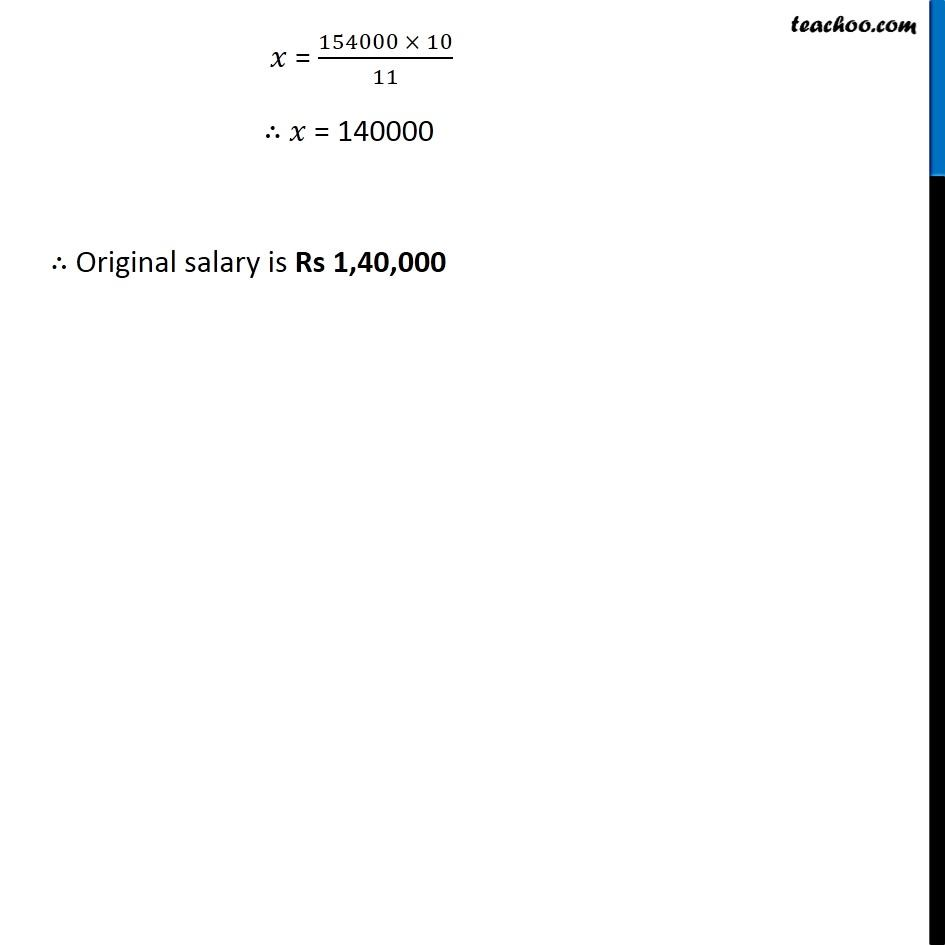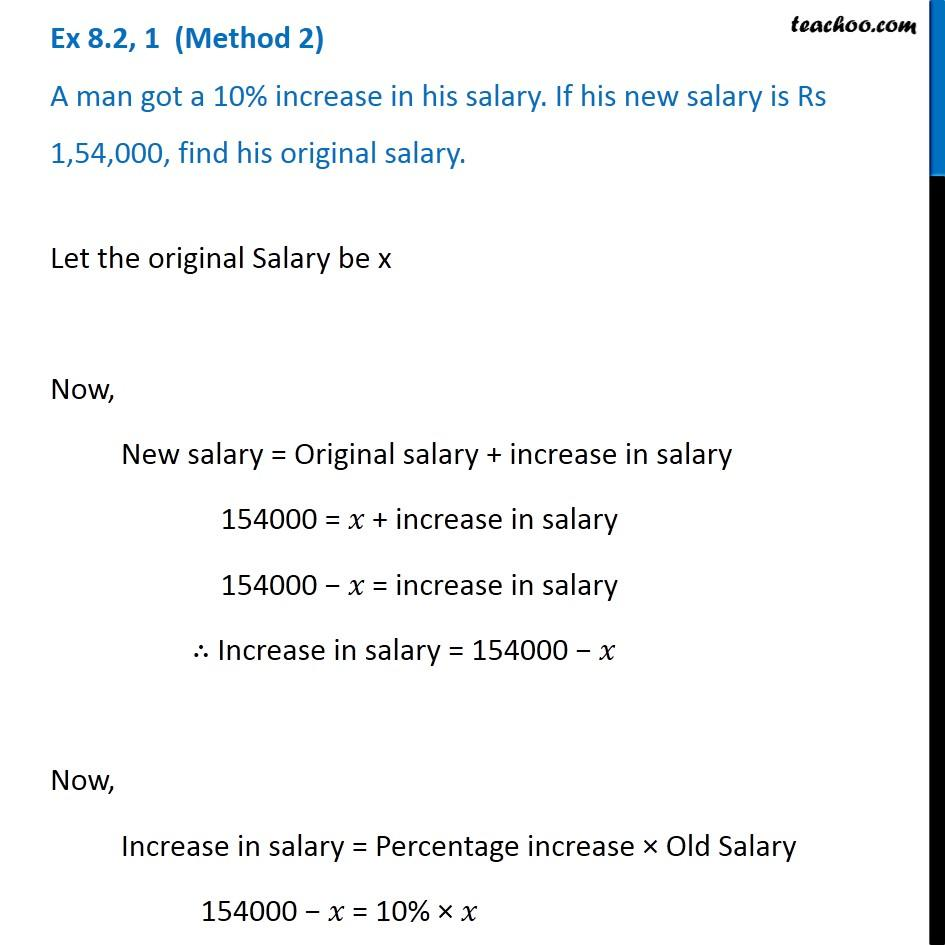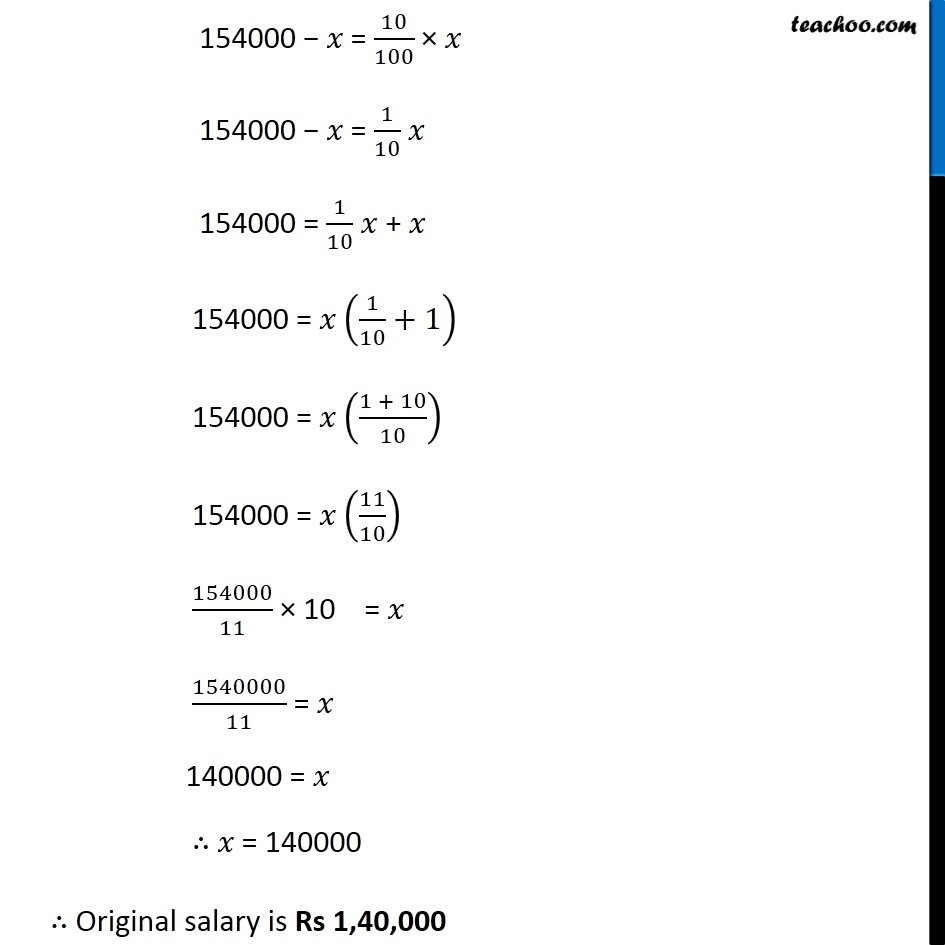Solve all your doubts with Teachoo Black (new monthly pack available now!)

### Transcript

Ex 8.2, 1 (Method 1) A man got a 10% increase in his salary. If his new salary is Rs 1,54,000, find his original salary. Given, Increase Percentage = 10% New salary = 154000 Let the original salary be x Now, New salary = Original salary + Increase in salary 154000 = x + Increase in salary 154000 − x = Increase in salary Increase in salary = 154000 − x Now, Percentage increase = (𝐼𝑛𝑐𝑟𝑒𝑎𝑠𝑒 𝑖𝑛 𝑠𝑎𝑙𝑎𝑟𝑦)/(𝑂𝑟𝑖𝑔𝑖𝑛𝑎𝑙 𝑠𝑎𝑙𝑎𝑟𝑦) × 100 10 = (154000 − 𝑥)/𝑥 × 100 10x = (154000 − 𝑥) × 100 10𝑥/100 = 154000 − 𝑥 𝑥/10 = 154000 𝑥/10 + 𝑥 = 154000 𝑥 (1/10+1) = 154000 𝑥 ((1 + 10)/10) = 154000 𝑥(11/10) = 154000 𝑥 = (154000 × 10)/11 ∴ 𝑥 = 140000 ∴ Original salary is Rs 1,40,000 Ex 8.2, 1 (Method 2) A man got a 10% increase in his salary. If his new salary is Rs 1,54,000, find his original salary.Let the original Salary be x Now, New salary = Original salary + increase in salary 154000 = 𝑥 + increase in salary 154000 − 𝑥 = increase in salary ∴ Increase in salary = 154000 − 𝑥 Now, Increase in salary = Percentage increase × Old Salary 154000 − 𝑥 = 10% × 𝑥 154000 − 𝑥 = 10/100 × 𝑥 154000 − 𝑥 = 1/10 𝑥 154000 = 1/10 𝑥 + 𝑥 154000 = 𝑥 (1/10+1) 154000 = 𝑥 ((1 + 10)/10) 154000 = 𝑥 (11/10) 154000/11 × 10 = 𝑥 1540000/11 = 𝑥 140000 = 𝑥 ∴ 𝑥 = 140000 ∴ Original salary is Rs 1,40,000